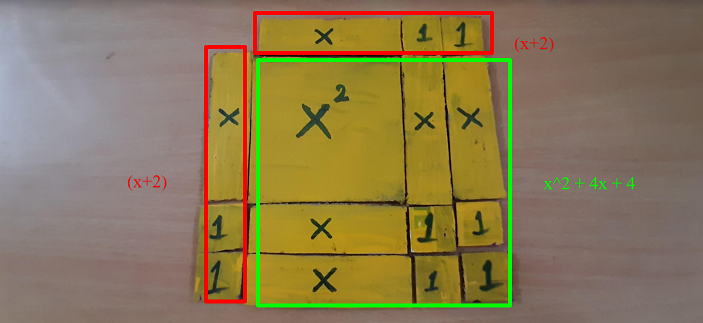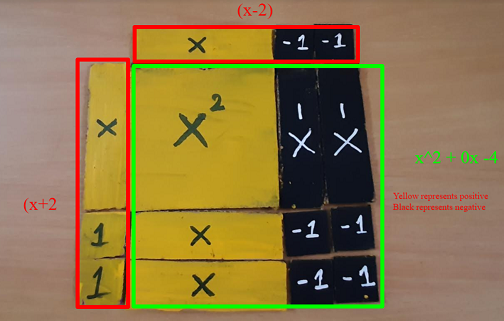# Algebraic kit

-Logeshwari, Tamizharasan

During the session with Ravi Alungati, we made a lot of math materials.

By using the cardboard boxes Gurumoorthy started doing Algebraic kit. Initially, I supported him. Then after that, I worked with Tamizharasan. We made x power 2, x and ones using the cardboard in inches.

To visually see a quadratic equation we can use this kit. The yellow-colored part is positive and the black colored part is negative. The squares are x power 2. The rectangle pieces are x and the small squares are the ones.

The example of a quadratic equation is as follows.

x^2 + 4x + 4 which can be factorized and we have (x+2) and (x+2)

The above equation can be represented as follows.